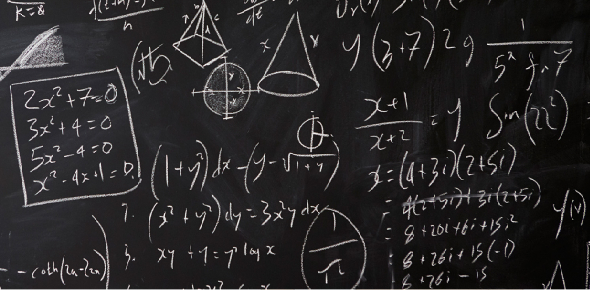Polynomial Division

3 QuestionsSettingsHow much do you actually know? This quiz will help you make sure you understand polynomial division.

• 1.
Which of these is a polynomial?
• A.

3

• B.

3x+9

• C.

-37829

• 2.
After the dividend is divided by the divisor, the left over is the
• 3.
When                                 15z3 – z2 -11z-3                                               3z2-2z-1What is the first term of the quotient?
• A.

3z

• B.

15

• C.

5z

• D.

X

Related Topics